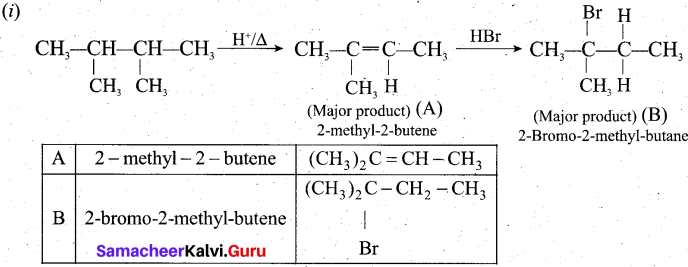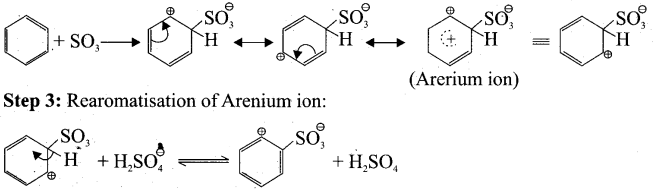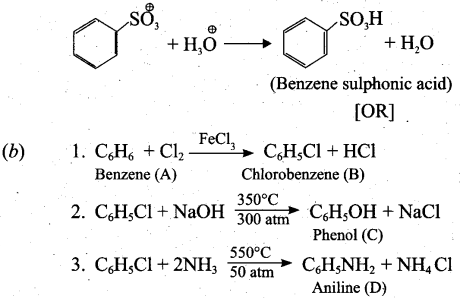Students can Download Tamil Nadu 11th Chemistry Model Question Paper 4 English Medium Pdf, Tamil Nadu 11th Chemistry Model Question Papers helps you to revise the complete Tamilnadu State Board New Syllabus and score more marks in your examinations.

## TN State Board 11th Chemistry Model Question Paper 4 English MediumTime: 2½ Hours
Total Score: 70 Marks

General Instructions:

1. The question paper comprises of four parts.
2. You are to attempt all the parts. An internal choice of questions is provided wherever applicable.
3. All questions of Part I. II, III and IV are to be attempted separately.
4. Question numbers 1 to 15 in Part I are Multiple Choice Questions of one mark each. These are to be answered by choosing the most suitable answer from the given four alternatives and writing the option code and the corresponding answer.
5. Question numbers 16 to 24 in Part II are two-mark questions. These are to be answered in about one or two sentences.
6. Question numbers 25 to 33 in Part III are three-mark questions. These are to be answered in about three to five short sentences.
7. Question numbers 34 to 38 in Part IV are five-mark questions. These are to be answered in detail. Draw diagrams wherever necessary.PART -1

Answer all the questions. [15 x 1 = 15]
Choose the most suitable answer from the given four alternatives.

Question 1.
Carbon forms two oxides, namely carbon monoxide and carbon dioxide. The equivalent mass of which element remains constant?
(a) Carbon
(b) Oxygen
(c) Both carbon and oxygen
(d) Neither carbon nor oxygen
(b) Oxygen

Reaction 1 : 2 C + O2 → 2 CO
2 x 12 g carbon combines with 32 g ofoxygen.
Hence, Equivalent mass of carbon = $$\frac{2 \times 12}{32}$$ x 8 = 6

Reaction 2 : C + O2 → CO2
12 g carbon combines with 32 g of oxygen.
Hence, Equivalent mass of carbon = $$\frac{12}{32}$$ x 8 = 3Question 2.
Electronic configuration of species M2+ is Is2 2s2 2p6 3s2 3p6 3d6 and its atomic weight is 56.
The number of neutrons in the nucleus of species M is ………………… .
(i) 26
(b) 22
(c) 30
(iv) 24
(c) 30

Solution:
M2+ : 1s2 2s2 2p6 3s2 3p6 3d6 ; M : Is2 2s2 2p6 3s2 3p6 3d8
Atomic number = 26
Mass number = 56
No. of neutrons = 56 – 26 = 30

Question 3.
Assertion: Helium has the highest value of ionization energy among all the elements known Reason: Helium has the highest value of electron affinity among all the elements known
(a) Both assertion and reason are true and reason is correct explanation for the assertion
(b) Both assertion and reason are true but the reason is not the correct explanation for the assertion
(c) Assertion is true and the reason is false
(d) Both assertion and the reason are false
(c) Assertion is true and the reason is falseQuestion 4.
At room temperature normal hydrogen consists of
(a) 25% ortho form + 75% para form
(b) 50% ortho form + 50% para form
(c) 75% ortho form + 25% para form
(d) 60% ortho form + 40% para form
(c) 75% ortho form + 25% para form

Question 5.
Match the following.
List-I List-II
A. Beryllium – 1. Sacrificial anode
B. Calcium – 2. X-ray tube radiation window
C. Magnesium – 3. Scavenger to remove oxygen in TV
D. Barium – 4. Getter in vacuum tubes
Code: A B C D
(a) 4 2 3 1
(b) 2 4 1 3
(c) 3 1 4 .2
(d) 1 3 2 4
(b) 2 4 1 3Question 6.
Which of the following pair will diffuse at the same rate?
(a) CO2 and N2O
(b) CO2 and NO
(c) CO2 and CO
(d) N2O and NO
(a) CO2 and N2O

Question 7.
An ideal gas expands from the volume of 1 x 10-3 m3 to 1 x 10-2 m3 at 300K against a constant pressure at 1 x 103 Nm-2. The work done is ………………… .
(a) -900 J
(b) 900 kJ
(c) 270 kJ
(d) -900 kJ
(a) -900 J

w = PΔV
w = – (1 x 105 Nm-2) (1 x 10-2 m3 – 1 x 10-3 m3)
w = 105 (10-2 – 10-3) Nm
w = 105(10 – 1) 10-3) J
w = 105 (9 x 10-3) J
w = 9 x 102 J
w = -900 JQuestion 8.
At a given temperature and pressure, the equilibrium constant values for the equilibria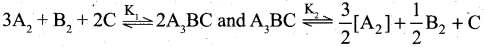The reaction between K1 and K2 is …………………. .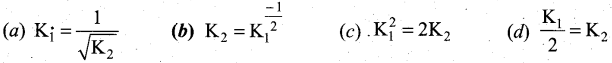b

Solution: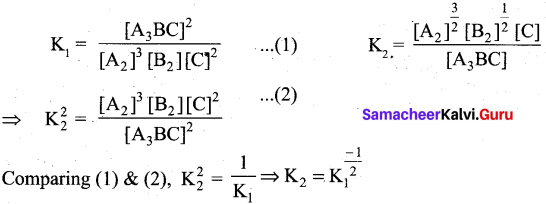Question 9.
Consider the following statements.
(i) Henry’s law is applicable at moderate temperature and pressure only.
(ii) Highly soluble gases obey’s Henry’s law.
(iii) The gases react with the solvent do not obey Henry’s law.
Which of the above statements is/are not correct?
(a) (i) only
(b) (ii) only
(c) (iii) only
(d) (i) and (ii)
(b) (ii) only

Question 10.
Which one of the following is the likely bond angles of sulphur tetrafluoride molecule?
(a) 120°, 80°
(b) 109°.28
(c) 90°
(d) 89°, 117°
(d) 89°, 117°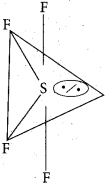Solution:
Normal bond angle in regular trigonal bipyramidal are 90° and 120°. Due to l.p – b.p repulsion, bond angle is reduced to 89°, 117° option (d).

Question 11.
The method used to estimate nitrogen in foods and fertilisers is
(a) Dumas method
(b) Kjeldahl’s method
(c) Carius method
(d) Oxide method
(b) Kjeldahl’s method

Question 12.
Which of the following carbocation will be most stable?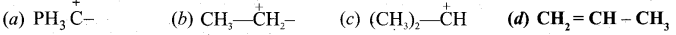dQuestion 13.
Which one of the following is used as a soil sterilizing agent?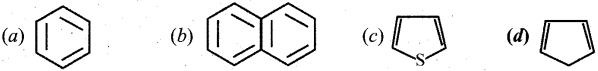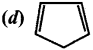Question 14.
Which of the following pair of oxides is responsible for acid rain?
(a) Chloroform
(b) Chloral
(c) Iodoform
(d) Chloropicrin
(d) Chloropicrin

Question 15.
Which of the following pair of oxides is responsible for acid rain?
(a) SO3 + NO2
(b) CO2 + CO
(c) N2O + CH4
(d) O2 + H2
(a) SO3 + NO2PART- II

Answer any six questions.in which question No. 20 is compulsory.

Question 16.
Calculate the number of moles present in 9g of ethane?
Mass of ethane = 9 g
Molar mass of ethane C2H6 = 30 g mol-1.
$$\text { No. of moles }=\frac{\text { Mass }}{\text { Molar mass }}=\frac{9}{30}=0.3 \mathrm{mol}$$

Question 17.
Why did halogens act as oxidizing agents?
Halogens act as oxidizing agents. Their electronic configuration is ns2 np5. So all the halogens are ready to gain one electron to attain the nearest inert gas configuration. An oxidizing agent is the one which is ready to gain an electron. So all the halogens act as oxidizing agents. Also halogens are highly electronegative with low dissociation energy and high negative electron gain enthalpies. Therefore, the halogens have a high tendency to gain an electron. Hence they act as oxidizing agents.Question 18.
Would it be easier to drink water with a straw on the top of Mount Everest?
It is difficult to drink water with a straw on the top of Mount Everest. This is because the reduced atmospheric pressure is less effective in pushing water into the straw at the top of the mountain because gravity falls off gradually with height. The air pressure falls off, there isn’t enough atmospheric pressure to push the water up in the straw all the way to the mouth.

Question 19.
Be(OH)2 is amphoteric in nature. Prove it.
Be(OH)2 is amphoteric in nature as it reacts with both acid and alkali.

• Be(OH)2 + 2NaOH → Na2BeO2 + 2H2O
(Acidic)
• Be(OH)2 + 2HC1 → BeCl2 + 2H2O
(Basic)

Question 20.
An engine operating between 127°C and 47°C takes some specified amount of heat from a high temperature reservoir. Calculate the percentage efficiency of an engine.
Given: T1 = 127°C = 127 + 273 = 400K
T2 = 47°C = 47 + 273 = 320 K
% efficiency q?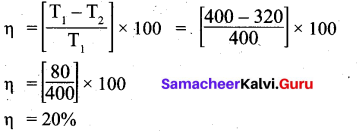Question 21.
Calculate the formal charge on each atom of carbonyl chloride (COCl2)?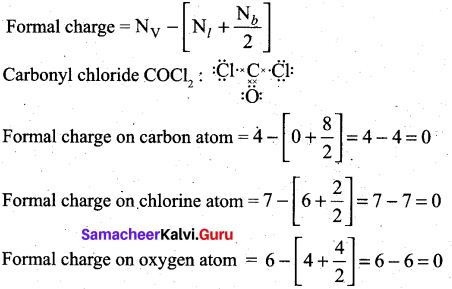Question 22.
Why we need to purify the organic compounds?
In order to study the structure, physical properties, chemical properties and biological properties of organic compounds, they must be in the pure state. So organic compounds must be purified.Question 23.
What are electrophiles? Give an example.
Electrophiles: Electrophiles are reagents that are attracted towards negative charge or electron rich center. They are either positively charged ions or electron deficient neutral molecules.

Example:
CO2, A1Cl3, BF3, FeCl3, NO+, NO2+, etc.

Question 24.
Complete the reaction, C2H5NH2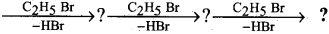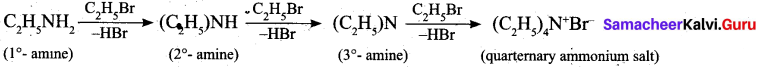Part – III

Answer any six questions in which question No. 30 is compulsory. [6 x 3 = 18]

Question 25.
On the formation of SF6 by the direct combination of S and F2, which is the limiting reagent? Prove it.
SF6 is formed by burning Sulphur in an atmosphere of Fluorine. Suppose 3 moles of S is allowed to react with 12 moles of Fluorine.
$$\mathrm{S}_{(\mathrm{o}}+3 \mathrm{F}_{2_{(\mathrm{g})}} \rightarrow \mathrm{SF}_{6(\mathrm{g})}$$As per the stoichiometric reaction, one mole of S reacts with 3 moles of fluorine to complete the reaction. Similarly, 3 moles of S requires only 9 moles of fluorine.

∴ It is understood that the limiting reagent is Sulphur and the excess reagent is Fluorine.

Question 26.
Explain about the significance of de-Broglie equation.

• $$\lambda=\frac{h}{m v}$$ This equation implies that a moving particle can be considered as a wave and a wave
can exhibit the properties of a particle.
• For a particle with high linear momentum (mv) the wavelength will be so small and cannot be observed.
• For a microscopic particle such as an electron, the mass is of the order of 10 31 kg, hence the wavelength is much larger than the size of atom and it becomes significant.
• For the electron, the de Broglie wavelength is significant and measurable while for the iron ball it is too small to measure, hence it becomes insignificant.

Question 27.
What are all the factors that influences electron affinity?
Factors that affects electron affinities are size of the atom, effective nuclear charge, screening effect.

• $$Electron affinity \propto \frac{1}{\text { Size of the atom }}$$
• Electron affinity QC Effective nuclear charge
• $$Electron affinity \propto \frac{1}{\text { Screening effect }}$$Question 28.
Describe about the biological importance of sodium and potassium.

• Monovalent sodium and potassium ions are found in large proportions in biological fluids.
• These ions perform important biological functions such as maintenance of ion balance and nerve impulse conduction.
• Sodium – Potassium play an important role in transmitting nerve signals.
• A typical 70 kg man has 90 g of Na and 170 g of K.
• Sodium ions are found on the outside of cells, being located in blood plasma and in the interstitial fluid which surrounds the cells. These ions participate in the transmission of nerve signals, in regulating the flow of water across cell membranes and in the transport of sugars and amino acids into cells.
• Potassium ions are the most abundant cations within cell fluids, where they activate many enzymes, participate in the oxidation of glucose to produce ATP and with sodium, are responsible for the transmission of nerve signals.

Question 29.
What are the conventions adopted in writing the thermochemical equation?
A thermochemical equation is a balanced stoichiometric chemical equation that includes the enthalpy change (ΔH).

• The coefficients in a balanced thermochemical equation refer to number of moles of reactants and products involved in the reaction.
• The enthalpy change of the reaction ΔH has unit kJ.
• When the chemical reaction is reversed, the value of ΔH is reversed in sign with the same magnitude.
• Physical states (gas, liquid, aqueous and solid) of all species is important and must be specified in a thermochemical reaction since ΔH depends on the phases of reactants and products.
• If the thermochemical equation is multiplied throughout by a number, the enthalpy change is also be multiplied by the same number value.
• The negative sign of $$\Delta \mathrm{H}_{r}^{o}$$ indicates the reaction to be an exothermic and the positive sign of $$\Delta \mathrm{H}_{r}^{o}$$ indicates an endothermic type of reaction.Question 30.
Calculate the work done when 2-moles of an ideal gas expands reversibly and isothermally from a volume of 500 mL to a volume 2 L at 25°C and normal pressure.
Given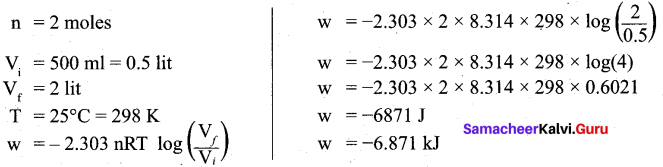Question 31.
For a gaseous homogeneous reaction at equilibrium number of moles of products are greater than the number of moles of reactants. Is K is larger or smaller than Kr.
For a homogeneous reaction at equilibrium, number of moles of products (np) are greater than the number of moles of reactants (nR), then Δng = +ve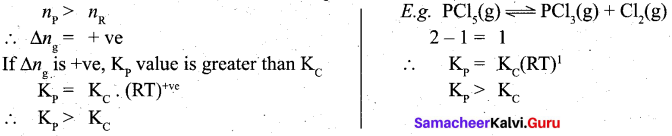Question 32.
Explain – Resonance.
(i) Certain organic compounds can be represented by more than one structure and they differ only in the position of bonding and lone pair of electrons. Such structures are called resonance structure and this phenomenon is called as resonance.This phenomenon is also called as mesomerism or mesomeric effect.
(ii) For example, the structure of aromatic compounds such as benzene and conjugated system like 1,3 butadiene cannot be represent by a single structure and their observed properties can be explained on the base of a resonance hybrid.
(iii) Resonance structure of benzene.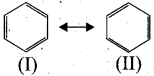(I) and (II) are called, as resonance hybrids of benzene.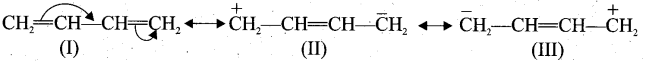(I), (II) and (III) are called as resonance hybrids of 1,3 butadiene.

Question 33.
Toluene undergoes nitration easily than benzene. Why?

• Toluene has a methyl group on the benzene ring which is electron releasing group and hence activate the benzene ring by pushing the electrons on the benzene ring.
• CH3 group is ortho – para director and ring activator. Therefore in toluene, ortho and para positions are the most reactive towards an electrophile, thus promoting electrophilic substitution reaction.
• The methyl group hence makes it around 25 times more reactive than benzene. Therefore it undergoes nitration easily than benzene.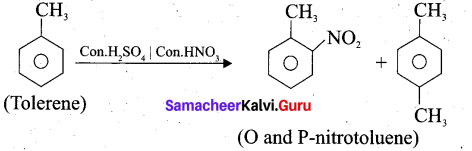Part – IV

Answer all the questions. [5 x 5 = 25]

Question 34.
(a) (i) Explain about the classification of matter. (3)
(ii) What is a combination reaction? Give an example. (2)
[OR]
(b) (i) Define – electronegativity. (2)
(ii) How Moseley determined the atomic number of an element using X-rays. (3)
(a) (i) Classification of matter: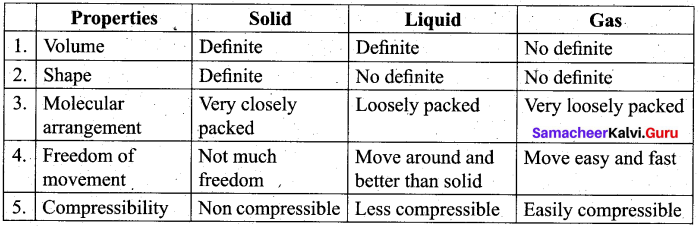(ii) When two or more substances combine to form a single substance, the reactions are called combination reactions.
A + B → C
Example: 2 Mg + O2 → 2MgO
[OR]
(b) (i) Electronegativity is the relative tendency of an element present in a covalently bonded molecule, to attract the shared pair of electrons towards itself.(ii)

• Henry Moseley studied the X-ray spectra of several elements and determined their atomic numbers (Z).
• He discovered a correlation between atomic number and the frequency of X-rays generated by bombarding an element with high energy of electrons.
• Moseley correlated the frequency of the X-ray emitted by an equation as, $$\sqrt{\mathfrak{v}}=a(\mathrm{Z}-b)$$
Where u = Frequency of the X-rays emitted by the elements. a and. b = Constants.
• From the square root of the measured frequency of the X-rays emitted, he determined the atomic number of the element.

Question 35.
(a) Explain the following observations,
(i) Aerated water bottles are kept under water during summer. (3)
(ii) Liquid ammonia bottle is cooled before opening the seal. (2)
[OR]
(b) Derive the relation between ΔH and ΔU for an ideal gas. (5)
(a) (i) In aerated water bottles, CO2 gas is passed through the aqueous solution under pressure because the solubility of the gas in water is not very high. In summer, the solubility of the gas in water is likely to decrease because of the rise in temperature. Thus, in summer, more ’ of gas will be present above the liquid surface in the glass bottle. In case, the pressure of the gas becomes too high, the glass will not be able to withstand the pressure and the bottle may explode. To avoid this, the bottles are kept under water. As a result, the temperature is likely to decrease and the solubility of CO2 is likely to increase in aqueous solution resulting in decreased pressure.(ii) Liquid ammonia bottle contains the gas under very high pressure. If the bottle is opened as such, then the sudden decrease in pressure will lead to a large increase in volume of the gas. As a result, the gas will come out of the bottle all of a sudden with force. This will lead to the breakage of the bottle and also causes accident However, if the bottle is cooled under tap water for sometime, there will be a decrease in the volume of a gas to a large extent. If the seal is opened now, the gas will come out of the bottle at a slower rate, reduces the chances of accident.
[OR]
(b) Relation between ΔH and ΔU for an ideal gas.
1. When the system at constant pressure undergoes changes from an initial state with Hp U1, V1 and P parameters to a final state with H2, U2, V2 and P parameters, the change in enthalpy AH, is given by
∆H = U + PV
2. At initial state H1 = U1 + PV1 ……………….. (1)
At final state H2 = U2 + PV2 ……………….. (2)
(2) – (1) ⇒ (H2 – H1) = (U2 – U1) + P (V2 – V1)
∆H = ∆U + P∆V …………………… (3)
Considering ∆U = q + w ; w = – P∆V
∆H = q + w + PAV
∆H = qp – PAV+ PAV
∆H = qp ………………… (4)qp is the heat absorbed at constant pressure and is considered as heat content.

3. Consider a closed system of gases which are chemically reacting to produce product gases at constant temperature and pressure with V. and Vf as the total volumes of the reactant and product gases respectively, and n. and nf are the number of moles of gaseous reactants and products. Then,
For reactants: PVi = niRT
For products : P Vf = nf RT

Then considering reactants as initial state and products as final state,
P (Vf – Vi) = (nf – ni) RT
P∆V = ∆ng RT

We know
∆H = ∆U + P∆V
∆H = ∆U + ∆ng RT …………………. (5)

Question 36.
(a) (i) What are heterogenous equilibrium? Give an example. (2)
(ii) The atmospheric oxidation of NO 2NO(g) + O2(g) = 2NO2(g) was studied with initial pressure of 1-atm of NO and 1-atm of O2. At equilibrium partial pressure of oxygen is 0.52 atm. Calculate Kp of the reaction. (3)
[OR]
(b) (i) Explain the factors influencing the solubility of the solutes. (3)
(ii) Why the carbonated drinks are stored in a pressurised container? (2)
(a) (i) Heterogeneous equilibrium: If the reactants and products of a reaction in equilibrium are in different phases, then it is called as heterogeneous equilibrium.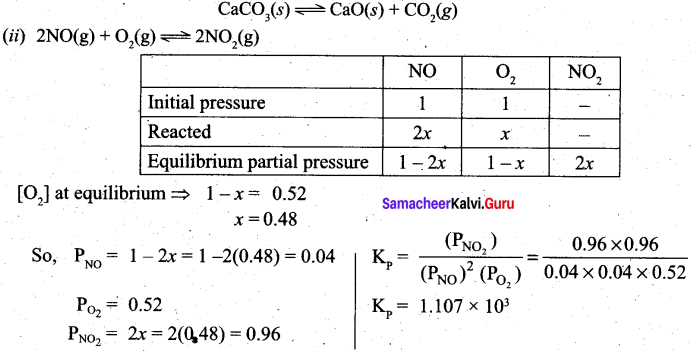(b) (i) Factors influencing solubility:
Nature of solute and solvent: Sodium chloride, an ionic compound readily dissolves in , polar solvent such as water but it does not dissolve in non polar solvent such as benzene. Most of the organic compounds dissolve in organic solvent and do not dissolve in water.

Effect of temperature: Generally, the solubility of a solid solute in a liquid solvent increases with increase in temperature. The dissolution of NaCl does not vary as the maximum solubility is achieved at normal temperature. The dissolution of ammonium nitrate is endothermic, the solubility increases with increase in temperature. The dissolution of ceric sulphate is exothermic and the solubility decreases with increase of temperature. In the case of gaseous solute in liquid solvent the solubility decreases with increase in temperature.

Effect of pressure: Generally the change in pressure does not have any significant effect in the solubility of solids and liquids as they are not compressible. However, the solubility of gases generally increases with increase of pressure.

(ii)

• The carbonated beverages contain CO2 dissolved in them. To dissolve the CO2 in these drinks, CO2 gas is bubbled through them under high pressure.
• These containers are sealed to maintain the pressure. When we open these containers at atmospheric pressure, the pressure of the CO2 drops to the atmospheric pressure level and hence bubbles of CO2 rapidly escape from the solution and show effervescence.Question 37.
(a) (i) Explain – paper chromatography. (3)
(ii) What are stereo-isomerism? (2)
[OR]
(b) (i) Explain the homolytic fission of a covalent bond? (3)
(ii) Why chloroacetic acid is more acidic than acetic acid? (2)
(a) (i) 1. It is an example of partition chromatography. A strip of paper acts as an adsorbent.
This method involves continues differential pardoning of components of a mixture between stationary and mobile phase. In paper chromatography, a special quality paper known as chromatographic paper is used. This paper act as a stationary phase.
2. A strip of chromatographic paper spotted at the base with the solution of the mixture is suspended in a suitable solvent which acts as the mobile phase. The solvent rises up and flows over the spot. The paper selectivity retains different components according to their different partition in the two phases where a chromatogram is developed.
3. The spots of the separated coloured components are visible at different heights from the position of initial spots on the chromatogram. The spots of the separated colourless compounds may be observed either under ultraviolet light or by the use of an appropriate spray reagent.

(ii) Stereo-isomerism: The isomers which have same bond connectivity but different arrangement of groups or atoms in space are known as stereoisomers. This phenomenon is known as stereoisomerism.

[OR](b) (i)

• Homolytic cleavage is the process in which a covalent bond breaks symmetrically in such way that fcach of the bonded atoms retains one electron.
• This type of cleavage occurs under high temperature or in the presence of UV light.
• In a compound containing non-‘polar covalent bond formed between atoms of similar electronegativity, in such molecules the cleavage of bonds results into free radicals.
• For example, ethane undergo homolytic fission to produce, two methyl free radicals.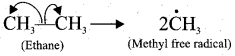(ii) Chloro acetic acid: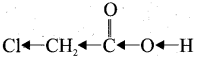Chloro acetic acid has Cl-group and it has high electronegativity and shows -I effect. Therefore Cl-atom to facilitate the dissociation of O-H bond very fastly. Whereas in the – case of acetic acid, has CH3 group and it shows +1 effect, therefore dissociation of O-H bond will be more difficult. Thus chloro acetic acid is stronger acid than acetic acid.Question 38.
(a)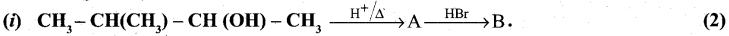Identify A and B (major products).
(ii) Describe the mechanism of sulphonation of benzene. (3)
[OR]
(b) An organic compounds A of a molecular formula C6H6 which is simple aromatic hydrocarbon. A reacts with Cl2 in presence of FeCl3 to give B. B reacts with NaOH at 350°C and 300 atm pressure to give C. B again reacts with ammonia at 250°C and 50 atm pressure to give D. Identify A, B, C and D explain the reaction. (5)